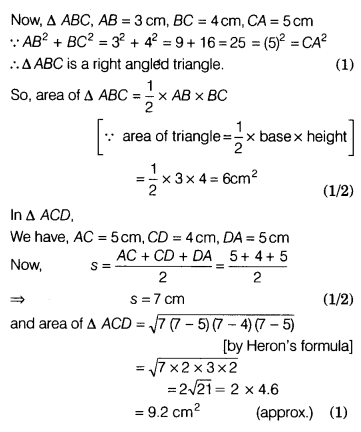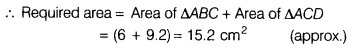# Find the area of a quadrilateral ABCD in which AB = 3 cm, BC = 4 cm, CD = 4 cm

Find the area of a quadrilateral ABCD in which AB = 3 cm, BC = 4 cm, CD = 4 cm, DA = 5 cm and AC = 5 cm.

Area of quadrilateral ABCD= Area of ∆ABC + Area of ∆ACD